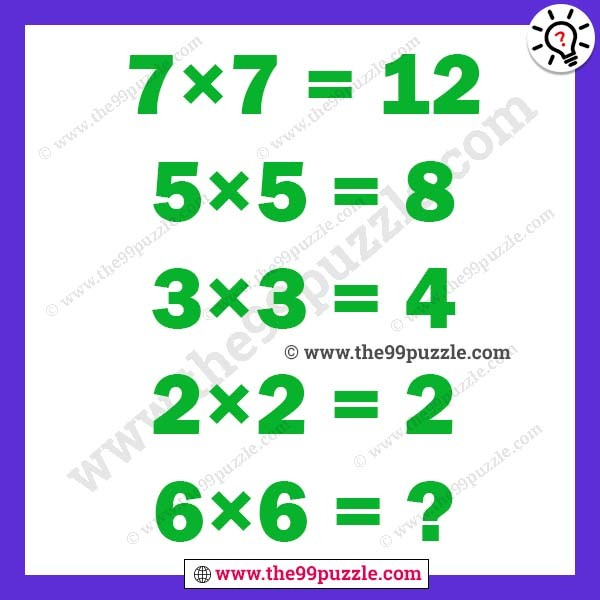# Only genius can solve math logic – Puzz143

Only genius can solve math logic. These types of puzzles can shake your brain. Observe this math puzzle and crack the math logic. If you are a genius solve the math logic last equation of this math puzzle.

7×7=12

5×5=8

3×3=4

2×2=2

6×6=?7×7=7+7−2=12

5×5=5+5−2=8

3×3=3+3−2=4

2×2=2+2−2=2

6×6=6+6−2=10